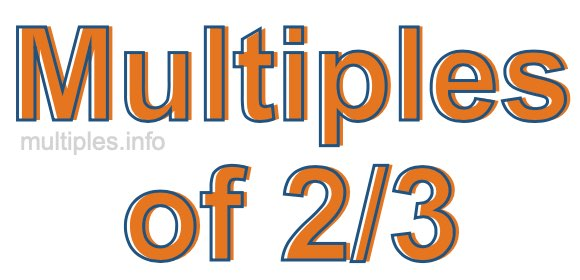Multiples of 2/3We define the multiples of 2/3 as the product of any integer multiplied by 2/3. Therefore, to create a list of multiples of 2/3 we start by multiplying 2/3 by one, then we multiply 2/3 by two, then we multiply 2/3 by three, and so on.

To multiply a fraction by an integer, we leave the denominator alone and multiply the numerator by the integer. It is impossible to give you a list of all multiples of 2/3 because the list goes on forever. However, here is the math to calculate the beginning list of multiples of 2/3.

2/3 × 1 = 2/3

2/3 × 2 = 4/3

2/3 × 4 = 6/3

2/3 × 4 = 8/3

2/3 × 5 = 10/3

2/3 × 6 = 12/3

2/3 × 7 = 14/3

2/3 × 8 = 16/3

2/3 × 9 = 18/3

2/3 × 10 = 20/3

2/3 × 11 = 22/3

2/3 × 12 = 24/3

We have simplified the fractions above for you. Below are the multiples of 2/3 in their lowest possible terms:

2/3 = 2/3

4/3 = 1 1/3

6/3 = 2

8/3 = 2 2/3

10/3 = 3 1/3

12/3 = 4

14/3 = 4 2/3

16/3 = 5 1/3

18/3 = 6

20/3 = 6 2/3

22/3 = 7 1/3

24/3 = 8

As we stated above, the list of multiples of 2/3 goes on forever because the product of 2/3 multiplied by any whole number (integer) is a multiple of 2/3. You can also create a list of multiples of 2/3 if you keep adding 2/3 like this:

2/3
2/3 + 2/3 = 4/3
2/3 + 2/3 + 2/3 = 6/3
2/3 + 2/3 + 2/3 + 2/3 = 8/3

Again, the list can go on forever, because you can continue adding 2/3 to the list. Therefore, the list of multiples of 2/3 is ∞ (infinite).

Multiples of Fractions
Do you need the multiples of another fraction? Enter a another fraction below to get the multiples of that fraction.

/

Multiples of 2/4
Go here for the next fraction on our list that we have multiples for.